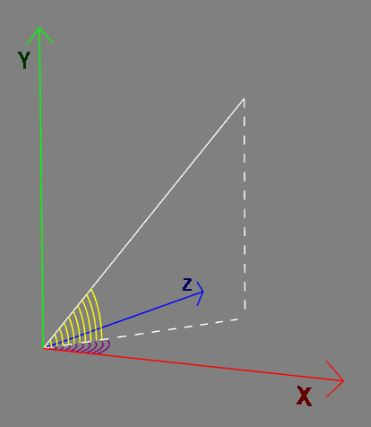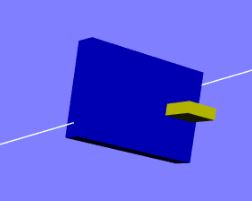# Design for Create Track

This page explains the design behind the 'createTrack' function which you use to build tracks that provide data for a carriage to follow. A track is built for an array of points (Vector3s) which is split into sections. Each section uses the designated lean, the rotation of the carriage about the track direction and turn, the rotation of the carriage about its local Y axis, of the carriage following the track to produce arrays of matrices for each point on the track path. The direction of a rail is given by the tilt (-π/2 to π/2) of the rail about the z axis and its swivel ( to to 2π) about the y axis.

## Rotation Matrices

Matrices are used since they are the fundamental operations needed to combine rotations in a given order. To produce the final rotation of a carriage at any point all the following rotations: tilt; swivel; lean; turn; need to be combined.track tilt (yellow angle) and swivel (purple angle)carriage lean and turn

## Obtaining the Track Data

The track data produce by createTrack are the arrays

• directions: directions of travel;
• rotations: a combination of the lean and turn rotations;
• carriageRotations: lean rotations;
• passengerRotations: turn rotations.

The parameters needed for each section are

• start index;

then the options

• lean;
• leanTwists;
• leanWaves;
• leanWaveAngle;
• turn;
• turnTwists;
• turnWaves;
• turnWaveAngle.

are used with

var section = new sectionData(startAt, options);

The code for which is

var sectionData = function (startAt, options) {  this.start = startAt;  this.options = options;};

This data for each section is passed as an array to 'createTrack' along with the points for the track as

var createTrack = function(points, sections) {

Internally 'createTrack' uses a 'createSection' function to construct the data to return.

## Section Build

The 'createSection' takes three parameters, points, the full points array for the track, the startSection which contains the information to be used in creating the section and endSection which just provides the lean and turn values at the end of the current section.

The term rail is used to indicate a segment of track from points[i] to points[i + 1]

function createSection(points, startSection, endSection) {  /***** Calculate the number of rails for the section *****/  var railsFrom = startSection.start; //index of start of section  var railsTo = endSection.start; //index of end of section  if (endSection.start === 0) {    railsTo = points.length;  }
var nbRails = railsTo - railsFrom;
/***** Set the defaults for the section *****/  var initialLean = startSection.options.lean === void 0 ? 0 : startSection.options.lean; //lean of carriage about direction axis at start, a phi variable  var initialTurn = startSection.options.turn === void 0 ? 0 : startSection.options.turn; // turn of carriage around upright at start, a theta variable  var leanTwists = startSection.options.leanTwists === void 0 ? 0 : startSection.options.leanTwists; //number of  lean twists (+ve counter clockwise, -ve clockwise)  var leanWaves = startSection.options.leanWaves === void 0 ? 0 : startSection.options.leanWaves; //number of lean waves  var leanWaveAngle = startSection.options.leanWaveAngle === void 0 ? 0 : startSection.options.leanWaveAngle; //angle for lean wave  var turnTwists = startSection.options.turnTwists === void 0 ? 0 : startSection.options.turnTwists; //number of  turn twists (+ve counter clockwise, -ve clockwise)  var turnWaves = startSection.options.turnWaves === void 0 ? 0 : startSection.options.turnWaves; //number of turn waves  var turnWaveAngle = startSection.options.turnWaveAngle === void 0 ? 0 : startSection.options.turnWaveAngle; //angle for turn wave
var finalLean = endSection.options.lean === void 0 ? 0 : endSection.options.lean;  var finalTurn = endSection.options.turn === void 0 ? 0 : endSection.options.turn;
/***** Check if both waves and  twists are used and ignore twists when so  *****/  //lean waves supersede lean twists unless leanWaveAngle = 0  if (leanWaves > 0 && Math.abs(leanTwists) > 0) {    if (leanWaveAngle == 0) {      leanWaves = 0;    } else {      leanTwists = 0;    }  }
//turn waves supersede turn twists unless turnWaveAngle = 0  if (turnWaves > 0 && Math.abs(turnTwists) > 0) {    if (turnWaveAngle == 0) {      turnWaves = 0;    } else {      turnTwists = 0;    }  }
/***** Initiate the variables and their types *****/  //rail transformation matrices for swivel and tilt rotations  var rotationMatrixY = BABYLON.Matrix.Identity();  var rotationMatrixZ = BABYLON.Matrix.Identity();  var rotationMatrix = BABYLON.Matrix.Identity();
var m = BABYLON.Matrix.Identity();  var initialRailDirection = BABYLON.Axis.X;  var initialUprightDirection = BABYLON.Axis.Y;  var initialLevelDirection = BABYLON.Axis.Z;  var railDirection = BABYLON.Vector3.Zero();  var uprightDirection = BABYLON.Vector3.Zero(); //contains vector normal to rail direction after tilt  var levelDirection = BABYLON.Vector3.Zero(); //contains vector normal to rail direction after swivel  var leanDirection = BABYLON.Vector3.Zero(); // contains vector normal to rail direction after tilt, swivel and lean  var turnDirection = BABYLON.Vector3.Zero();  var carriageNormal = BABYLON.Vector3.Zero();  var rotationMatrixLean = BABYLON.Matrix.Identity();  var rotationMatrixTurn = BABYLON.Matrix.Identity();  var rotationMatrixPassenger = BABYLON.Matrix.Identity();  var initialPosition = BABYLON.Vector3.Zero();  var rotation = BABYLON.Matrix.Identity(); //Overall rotation after tilt, swivel, lean and turn
var tilt = 0; //tilt angle of rail after rotation about (0, 0, 1)  var swivel = 0; //swivel angle of rail after rotation around (0, 1, 0)
var railCount = 0; // determines how many rails along a section, used to control wave values
//Set calculated values for variables  var phi = initialLean;  var theta = initialTurn;
var gradLean = (finalLean - initialLean) / (nbRails - 1); // lean gradient  var gradTurn = (finalTurn - initialTurn) / (nbRails - 1); // turn gradient
var deltaPhi = (finalLean + 2 * leanTwists * Math.PI - initialLean) / nbRails; //increase in phi per rail for lean twist  var deltaTheta = (finalTurn + 2 * turnTwists * Math.PI - initialTurn) / nbRails; //increase in theta per rail for lean twist
/***** Loop over rails in section*****/
for (let i = railsFrom; i < railsTo; i++) {    points[(i + 1) % points.length].subtractToRef(points[i], railDirection); //direction of each rail    railDirection.normalize();    swivel = -Math.atan2(railDirection.z, railDirection.x); //swivel angle rail makes in xz plane    tilt = Math.atan2(Math.abs(railDirection.y), Math.abs(railDirection.x)); // tilt angle rail makes in first quadrant of xy plane    tilt *= Math.sign(railDirection.y); //tilt angle of rail in xy plane from -pi/2 to pi/2    BABYLON.Matrix.RotationAxisToRef(BABYLON.Axis.Y, swivel, rotationMatrixY); //swivel angle to matrix rotation    BABYLON.Matrix.RotationAxisToRef(BABYLON.Axis.Z, tilt, rotationMatrixZ); //tilt angle to matrix rotation    rotationMatrixZ.multiplyToRef(rotationMatrixY, rotationMatrix); // form combined swivel and tilt matrix    BABYLON.Vector3.TransformNormalToRef(initialUprightDirection, rotationMatrix, uprightDirection); //swivel, tilt act on carriage upright    BABYLON.Vector3.TransformNormalToRef(initialLevelDirection, rotationMatrix, levelDirection); //swivel, tilt act on carriage level    uprightDirection.normalize();    levelDirection.normalize();
if (leanWaves > 0) {      phi = initialLean + railCount * gradLean + leanWaveAngle * Math.sin((railCount * leanWaves * Math.PI) / (nbRails - 1));    } else {      phi += deltaPhi;    }    if (turnWaves > 0) {      theta = initialTurn + railCount * gradTurn + turnWaveAngle * Math.sin((railCount * turnWaves * Math.PI) / (nbRails - 1));    } else {      theta += deltaTheta;    }    railCount++;    BABYLON.Matrix.RotationAxisToRef(railDirection, phi, rotationMatrixLean); // lean matrix    BABYLON.Vector3.TransformNormalToRef(uprightDirection, rotationMatrixLean, carriageNormal); // lean applied to upright    BABYLON.Matrix.RotationAxisToRef(carriageNormal, theta, rotationMatrixTurn); // turn applied to upright after lean
BABYLON.Matrix.RotationAxisToRef(initialUprightDirection, theta, rotationMatrixPassenger); //just turn applied to upright    passengerRotations.push(rotationMatrixPassenger.clone());
rotationMatrix.multiplyToRef(rotationMatrixLean, rotation); //rotation from swivel, tilt, and lean only    carriageRotations.push(rotation.clone());    rotation.multiplyToRef(rotationMatrixTurn, rotation); //complete rotation from swivel, tilt, lean and turn    rotations.push(rotation.clone());
directions.push(railDirection.clone());  }}

## Create Track from Sections

The createTrack function requires the points for the path of the track and an array of sections. Checking is carried out on the order of sections.

var createTrack = function (points, sections) {  //Data arrays  var directions = [];  var rotations = [];  var carriageRotations = [];  var passengerRotations = [];
var nbSections = sections.length;
var looped = sections[nbSections - 1].start === 0;  for (let i = 1; i < nbSections - looped; i++) {    if (sections[i - 1].start > sections[i].start) {      console.log("sections not in order");      return;    }  }  if (0 < sections[nbSections - 1].start && sections[nbSections - 2].start > sections[nbSections - 1].start) {    console.log("last section not in order");    return;  }  var section = sections;  if (section.start > 0) {    startSection = new sectionData(0, {});    sections.unshift(startSection);    nbSections = sections.length;  }
if (0 < sections[nbSections - 1].start && sections[nbSections - 1].start < points.length - 1) {    //assume need to close loop    var endSection = new sectionData(0, sections.options);    sections.push(endSection);  }
//Store track data per section  for (let i = 0; i < sections.length - 1; i++) {    createSection(points, sections[i], sections[i + 1]);  }
return { directions: directions, rotations: rotations, carriageRotations: carriageRotations, passengerRotations: passengerRotations };
/****** createSection function goes here *******/};

## Playground Example

PG: Set Carriage Variables